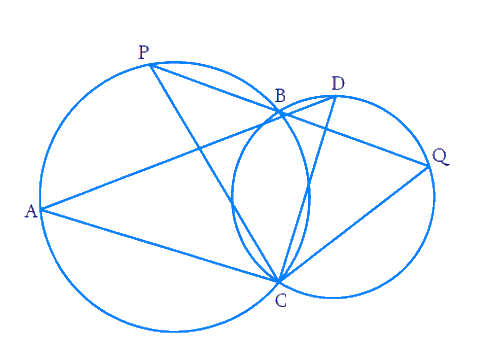# Ex.10.5 Q9 Circles Solution - NCERT Maths Class 9

Go back to  'Ex.10.5'

## Question

Two circles intersect at two points $${B}$$ and $${C.}$$ Through $${B,}$$ two line segments $${ABD}$$ and $${PBQ}$$ are drawn to intersect the circles at $$A, D, P$$ and $$Q$$ respectively. Prove that \begin {align} \angle {ACP}=\angle {QCD.} \end {align}Video Solution
Circles
Ex 10.5 | Question 9

## Text Solution

What is given ?

Two circles intersect at two points

What is unknown?

Proof of $$\angle {ACP}=\angle {QCD}$$

Reasoning:

$$\angle {ACP}$$  and  $$\angle {ABP}$$ lie on the same segment.

Similarly, $$\angle {DCQ}$$  and  $$\angle {DBQ}$$ lie on the same segment.

Angles in the same segment of a circle are equal.

Steps:

We know that, angles in the same segment of a circle are equal.

So we get  $$\angle {ACP}=\angle {ABP}$$  and  $$\angle {QCD}=\angle {QBD}$$

Also, \begin {align} \angle {QBD}=\angle {ABP} \end {align}(Vertically opposite angles)

Therefore $$\angle {ACP}=\angle {QCD}$$

Learn from the best math teachers and top your exams

• Live one on one classroom and doubt clearing
• Practice worksheets in and after class for conceptual clarity
• Personalized curriculum to keep up with school